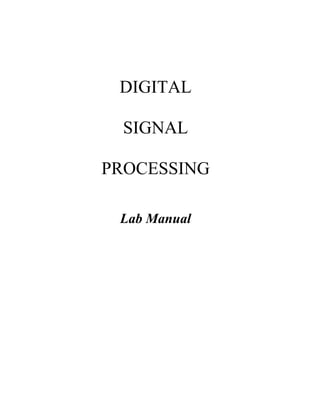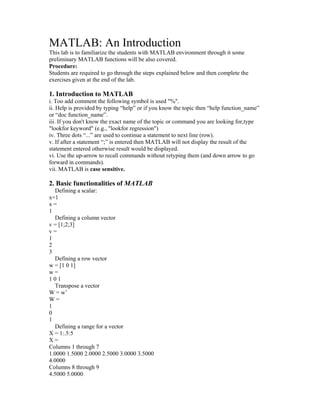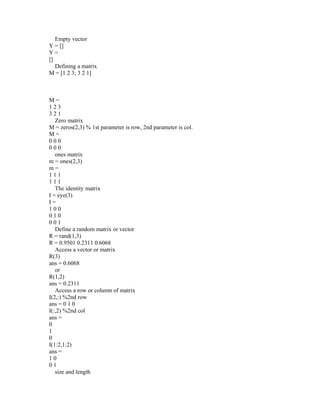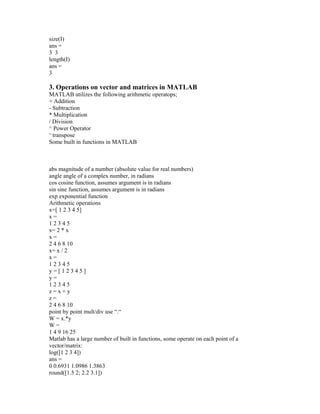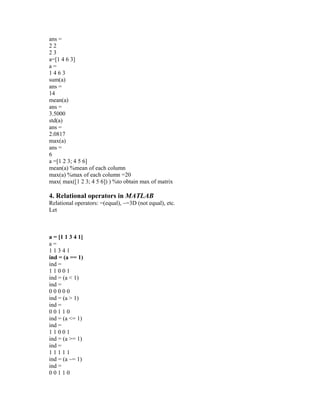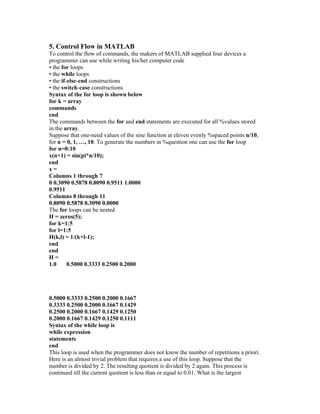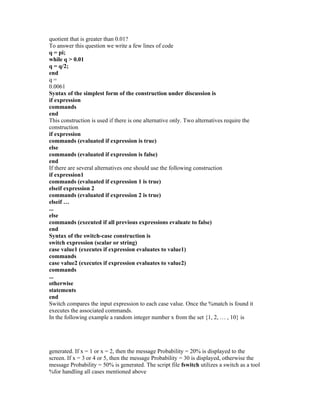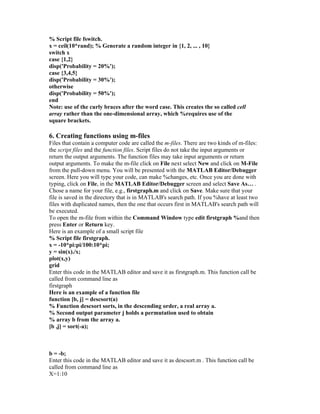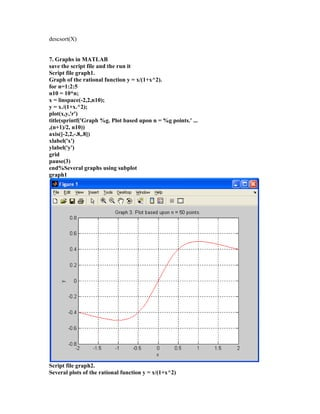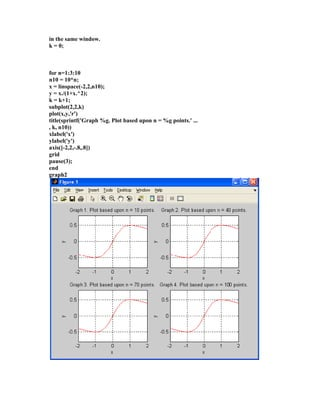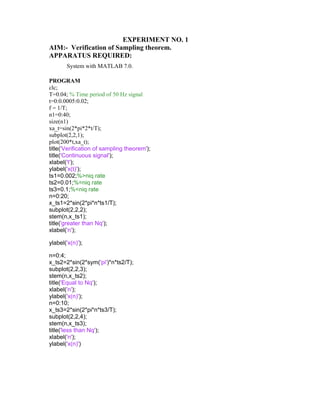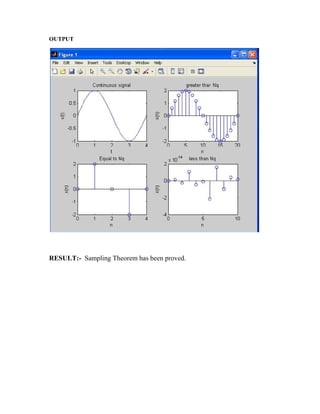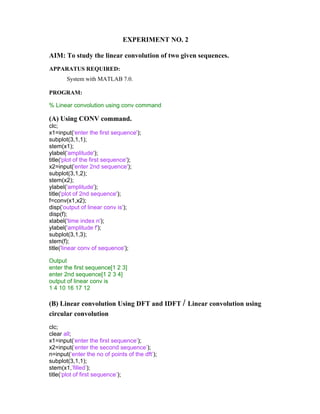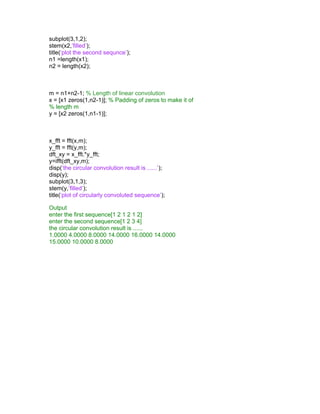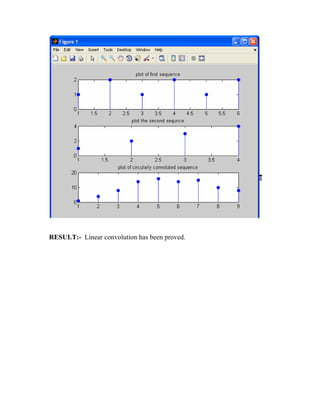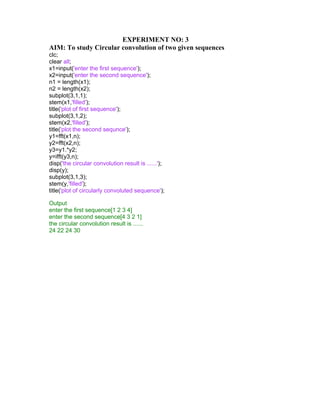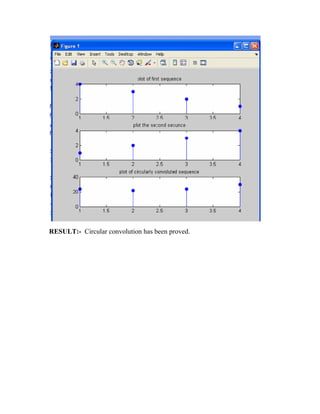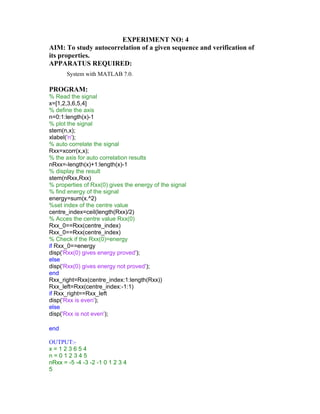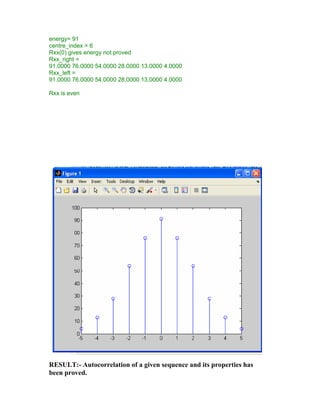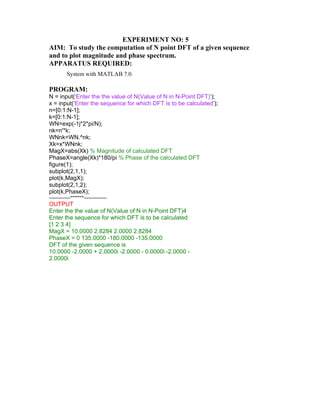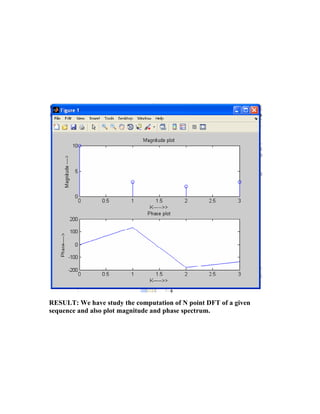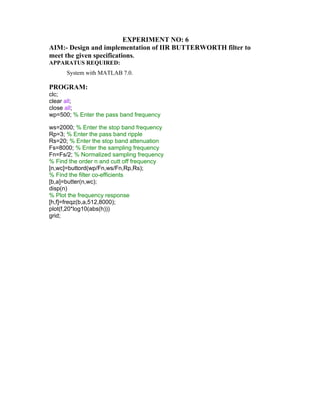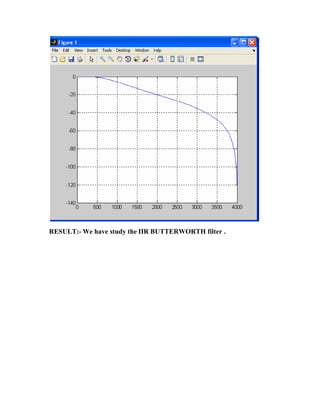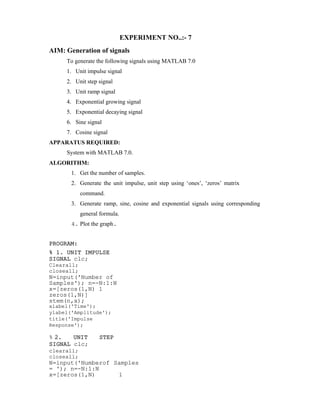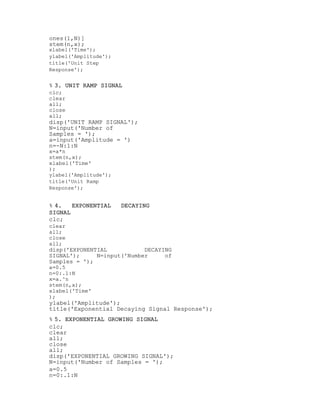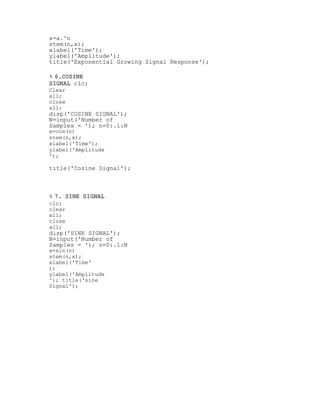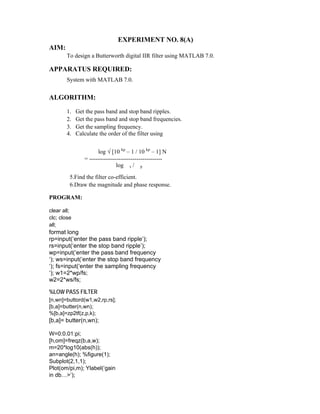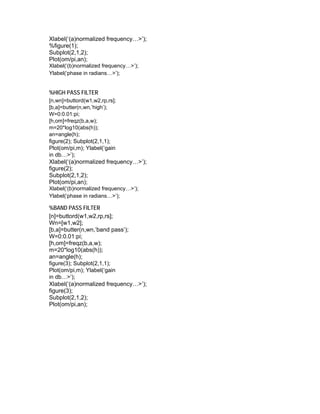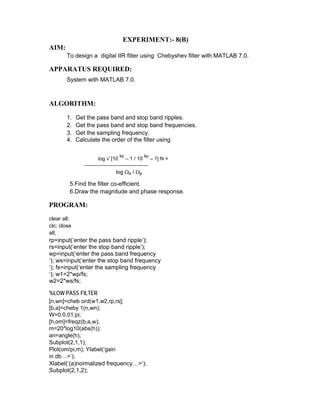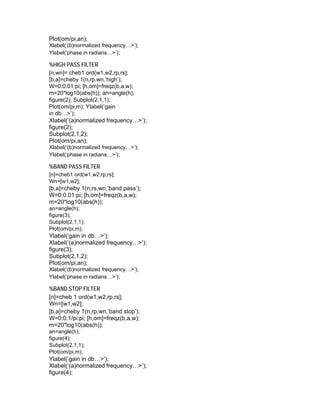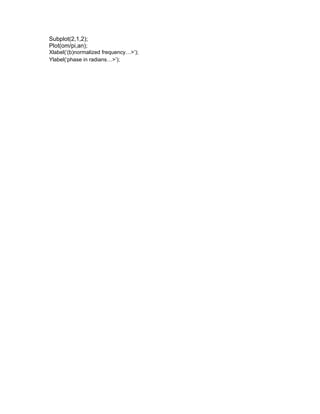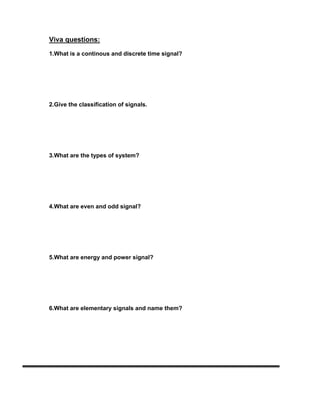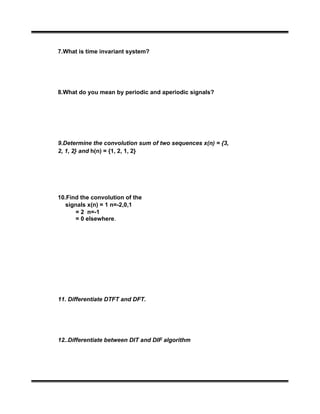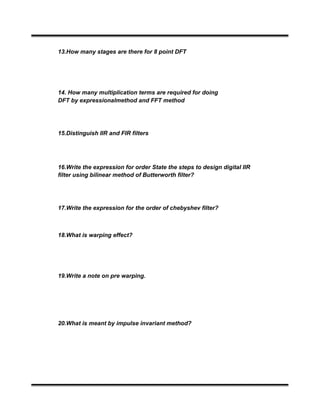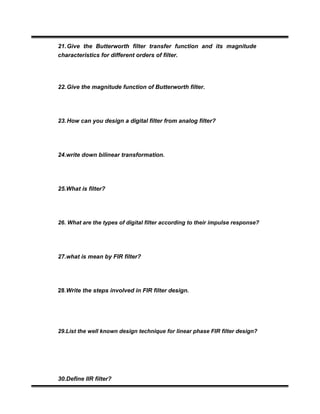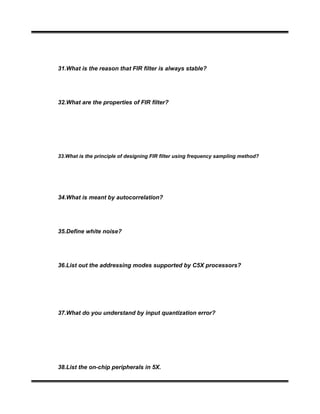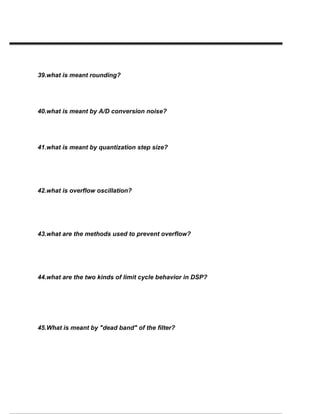Próximo SlideShare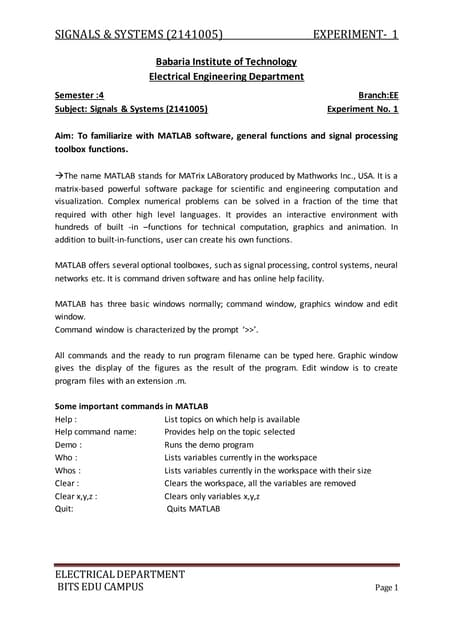signal and system
Cargando en ... 3
1 de 37

### Dsp lab _eec-652__vi_sem_18012013

1. DIGITAL SIGNAL PROCESSING Lab Manual
2. MATLAB: An Introduction This lab is to familiarize the students with MATLAB environment through it some preliminary MATLAB functions will be also covered. Procedure: Students are required to go through the steps explained below and then complete the exercises given at the end of the lab. 1. Introduction to MATLAB i. Too add comment the following symbol is used "%". ii. Help is provided by typing “help” or if you know the topic then “help function_name” or “doc function_name”. iii. If you don't know the exact name of the topic or command you are looking for,type "lookfor keyword" (e.g., "lookfor regression") iv. Three dots “...” are used to continue a statement to next line (row). v. If after a statement “;” is entered then MATLAB will not display the result of the statement entered otherwise result would be displayed. vi. Use the up-arrow to recall commands without retyping them (and down arrow to go forward in commands). vii. MATLAB is case sensitive. 2. Basic functionalities of MATLAB Defining a scalar: x=1 x = 1 Defining a column vector v = [1;2;3] v = 1 2 3 Defining a row vector w = [1 0 1] w = 1 0 1 Transpose a vector W = w’ W = 1 0 1 Defining a range for a vector X = 1:.5:5 X = Columns 1 through 7 1.0000 1.5000 2.0000 2.5000 3.0000 3.5000 4.0000 Columns 8 through 9 4.5000 5.0000
3. Empty vector Y = [] Y = [] Defining a matrix M = [1 2 3; 3 2 1] M = 1 2 3 3 2 1 Zero matrix M = zeros(2,3) % 1st parameter is row, 2nd parameter is col. M = 0 0 0 0 0 0 ones matrix m = ones(2,3) m = 1 1 1 1 1 1 The identity matrix I = eye(3) I = 1 0 0 0 1 0 0 0 1 Define a random matrix or vector R = rand(1,3) R = 0.9501 0.2311 0.6068 Access a vector or matrix R(3) ans = 0.6068 or R(1,2) ans = 0.2311 Access a row or column of matrix I(2,:) %2nd row ans = 0 1 0 I(:,2) %2nd col ans = 0 1 0 I(1:2,1:2) ans = 1 0 0 1 size and length
4. size(I) ans = 3 3 length(I) ans = 3 3. Operations on vector and matrices in MATLAB MATLAB utilizes the following arithmetic operatops; + Addition - Subtraction * Multiplication / Division ^ Power Operator ‘ transpose Some built in functions in MATLAB abs magnitude of a number (absolute value for real numbers) angle angle of a complex number, in radians cos cosine function, assumes argument is in radians sin sine function, assumes argument is in radians exp exponential function Arithmetic operations x=[ 1 2 3 4 5] x = 1 2 3 4 5 x= 2 * x x = 2 4 6 8 10 x= x / 2 x = 1 2 3 4 5 y = [ 1 2 3 4 5 ] y = 1 2 3 4 5 z = x + y z = 2 4 6 8 10 point by point mult/div use “.“ W = x.*y W = 1 4 9 16 25 Matlab has a large number of built in functions, some operate on each point of a vector/matrix: log([1 2 3 4]) ans = 0 0.6931 1.0986 1.3863 round([1.5 2; 2.2 3.1])
5. ans = 2 2 2 3 a=[1 4 6 3] a = 1 4 6 3 sum(a) ans = 14 mean(a) ans = 3.5000 std(a) ans = 2.0817 max(a) ans = 6 a =[1 2 3; 4 5 6] mean(a) %mean of each column max(a) %max of each column =20 max( max([1 2 3; 4 5 6]) ) %to obtain max of matrix 4. Relational operators in MATLAB Relational operators: =(equal), ~=3D (not equal), etc. Let a = [1 1 3 4 1] a = 1 1 3 4 1 ind = (a == 1) ind = 1 1 0 0 1 ind = (a < 1) ind = 0 0 0 0 0 ind = (a > 1) ind = 0 0 1 1 0 ind = (a <= 1) ind = 1 1 0 0 1 ind = (a >= 1) ind = 1 1 1 1 1 ind = (a ~= 1) ind = 0 0 1 1 0
6. 5. Control Flow in MATLAB To control the flow of commands, the makers of MATLAB supplied four devices a programmer can use while writing his/her computer code • the for loops • the while loops • the if-else-end constructions • the switch-case constructions Syntax of the for loop is shown below for k = array commands end The commands between the for and end statements are executed for all %values stored in the array. Suppose that one-need values of the sine function at eleven evenly %spaced points n/10, for n = 0, 1, …, 10. To generate the numbers in %question one can use the for loop for n=0:10 x(n+1) = sin(pi*n/10); end x = Columns 1 through 7 0 0.3090 0.5878 0.8090 0.9511 1.0000 0.9511 Columns 8 through 11 0.8090 0.5878 0.3090 0.0000 The for loops can be nested H = zeros(5); for k=1:5 for l=1:5 H(k,l) = 1/(k+l-1); end end H = 1.0 0.5000 0.3333 0.2500 0.2000 0.5000 0.3333 0.2500 0.2000 0.1667 0.3333 0.2500 0.2000 0.1667 0.1429 0.2500 0.2000 0.1667 0.1429 0.1250 0.2000 0.1667 0.1429 0.1250 0.1111 Syntax of the while loop is while expression statements end This loop is used when the programmer does not know the number of repetitions a priori. Here is an almost trivial problem that requires a use of this loop. Suppose that the number is divided by 2. The resulting quotient is divided by 2 again. This process is continued till the current quotient is less than or equal to 0.01. What is the largest
7. quotient that is greater than 0.01? To answer this question we write a few lines of code q = pi; while q > 0.01 q = q/2; end q = 0.0061 Syntax of the simplest form of the construction under discussion is if expression commands end This construction is used if there is one alternative only. Two alternatives require the construction if expression commands (evaluated if expression is true) else commands (evaluated if expression is false) end If there are several alternatives one should use the following construction if expression1 commands (evaluated if expression 1 is true) elseif expression 2 commands (evaluated if expression 2 is true) elseif … ... else commands (executed if all previous expressions evaluate to false) end Syntax of the switch-case construction is switch expression (scalar or string) case value1 (executes if expression evaluates to value1) commands case value2 (executes if expression evaluates to value2) commands ... otherwise statements end Switch compares the input expression to each case value. Once the %match is found it executes the associated commands. In the following example a random integer number x from the set {1, 2, … , 10} is generated. If x = 1 or x = 2, then the message Probability = 20% is displayed to the screen. If x = 3 or 4 or 5, then the message Probability = 30 is displayed, otherwise the message Probability = 50% is generated. The script file fswitch utilizes a switch as a tool %for handling all cases mentioned above
8. % Script file fswitch. x = ceil(10*rand); % Generate a random integer in {1, 2, ... , 10} switch x case {1,2} disp('Probability = 20%'); case {3,4,5} disp('Probability = 30%'); otherwise disp('Probability = 50%'); end Note: use of the curly braces after the word case. This creates the so called cell array rather than the one-dimensional array, which %requires use of the square brackets. 6. Creating functions using m-files Files that contain a computer code are called the m-files. There are two kinds of m-files: the script files and the function files. Script files do not take the input arguments or return the output arguments. The function files may take input arguments or return output arguments. To make the m-file click on File next select New and click on M-File from the pull-down menu. You will be presented with the MATLAB Editor/Debugger screen. Here you will type your code, can make %changes, etc. Once you are done with typing, click on File, in the MATLAB Editor/Debugger screen and select Save As… . Chose a name for your file, e.g., firstgraph.m and click on Save. Make sure that your file is saved in the directory that is in MATLAB's search path. If you %have at least two files with duplicated names, then the one that occurs first in MATLAB's search path will be executed. To open the m-file from within the Command Window type edit firstgraph %and then press Enter or Return key. Here is an example of a small script file % Script file firstgraph. x = -10*pi:pi/100:10*pi; y = sin(x)./x; plot(x,y) grid Enter this code in the MATLAB editor and save it as firstgraph.m. This function call be called from command line as firstgraph Here is an example of a function file function [b, j] = descsort(a) % Function descsort sorts, in the descending order, a real array a. % Second output parameter j holds a permutation used to obtain % array b from the array a. [b ,j] = sort(-a); b = -b; Enter this code in the MATLAB editor and save it as descsort.m . This function call be called from command line as X=1:10
9. descsort(X) 7. Graphs in MATLAB save the script file and the run it Script file graph1. Graph of the rational function y = x/(1+x^2). for n=1:2:5 n10 = 10*n; x = linspace(-2,2,n10); y = x./(1+x.^2); plot(x,y,'r') title(sprintf('Graph %g. Plot based upon n = %g points.' ... ,(n+1)/2, n10)) axis([-2,2,-.8,.8]) xlabel('x') ylabel('y') grid pause(3) end%Several graphs using subplot graph1 Script file graph2. Several plots of the rational function y = x/(1+x^2)
10. in the same window. k = 0; for n=1:3:10 n10 = 10*n; x = linspace(-2,2,n10); y = x./(1+x.^2); k = k+1; subplot(2,2,k) plot(x,y,'r') title(sprintf('Graph %g. Plot based upon n = %g points.' ... , k, n10)) xlabel('x') ylabel('y') axis([-2,2,-.8,.8]) grid pause(3); end graph2
11. EXPERIMENT NO. 1 AIM:- Verification of Sampling theorem. APPARATUS REQUIRED: System with MATLAB 7.0. PROGRAM clc; T=0.04; % Time period of 50 Hz signal t=0:0.0005:0.02; f = 1/T; n1=0:40; size(n1) xa_t=sin(2*pi*2*t/T); subplot(2,2,1); plot(200*t,xa_t); title('Verification of sampling theorem'); title('Continuous signal'); xlabel('t'); ylabel('x(t)'); ts1=0.002;%>niq rate ts2=0.01;%=niq rate ts3=0.1;%<niq rate n=0:20; x_ts1=2*sin(2*pi*n*ts1/T); subplot(2,2,2); stem(n,x_ts1); title('greater than Nq'); xlabel('n'); ylabel('x(n)'); n=0:4; x_ts2=2*sin(2*sym('pi')*n*ts2/T); subplot(2,2,3); stem(n,x_ts2); title('Equal to Nq'); xlabel('n'); ylabel('x(n)'); n=0:10; x_ts3=2*sin(2*pi*n*ts3/T); subplot(2,2,4); stem(n,x_ts3); title('less than Nq'); xlabel('n'); ylabel('x(n)')
12. OUTPUT   RESULT:- Sampling Theorem has been proved.
13. EXPERIMENT NO. 2 AIM: To study the linear convolution of two given sequences. APPARATUS REQUIRED: System with MATLAB 7.0. PROGRAM: % Linear convolution using conv command (A) Using CONV command. clc; x1=input('enter the first sequence'); subplot(3,1,1); stem(x1); ylabel('amplitude'); title('plot of the first sequence'); x2=input('enter 2nd sequence'); subplot(3,1,2); stem(x2); ylabel('amplitude'); title('plot of 2nd sequence'); f=conv(x1,x2); disp('output of linear conv is'); disp(f); xlabel('time index n'); ylabel('amplitude f'); subplot(3,1,3); stem(f); title('linear conv of sequence'); Output enter the first sequence[1 2 3] enter 2nd sequence[1 2 3 4] output of linear conv is 1 4 10 16 17 12 (B) Linear convolution Using DFT and IDFT / Linear convolution using circular convolution clc; clear all; x1=input(‘enter the first sequence’); x2=input(‘enter the second sequence’); n=input(‘enter the no of points of the dft’); subplot(3,1,1); stem(x1,’filled’); title(‘plot of first sequence’);
14. subplot(3,1,2); stem(x2,’filled’); title(‘plot the second sequnce’); n1 =length(x1); n2 = length(x2); m = n1+n2-1; % Length of linear convolution x = [x1 zeros(1,n2-1)]; % Padding of zeros to make it of % length m y = [x2 zeros(1,n1-1)]; x_fft = fft(x,m); y_fft = fft(y,m); dft_xy = x_fft.*y_fft; y=ifft(dft_xy,m); disp(‘the circular convolution result is ......’); disp(y); subplot(3,1,3); stem(y,’filled’); title(‘plot of circularly convoluted sequence’); Output enter the first sequence[1 2 1 2 1 2] enter the second sequence[1 2 3 4] the circular convolution result is ...... 1.0000 4.0000 8.0000 14.0000 16.0000 14.0000 15.0000 10.0000 8.0000
15.   RESULT:- Linear convolution has been proved.
16. EXPERIMENT NO: 3 AIM: To study Circular convolution of two given sequences clc; clear all; x1=input('enter the first sequence'); x2=input('enter the second sequence'); n1 = length(x1); n2 = length(x2); subplot(3,1,1); stem(x1,'filled'); title('plot of first sequence'); subplot(3,1,2); stem(x2,'filled'); title('plot the second sequnce'); y1=fft(x1,n); y2=fft(x2,n); y3=y1.*y2; y=ifft(y3,n); disp('the circular convolution result is ......'); disp(y); subplot(3,1,3); stem(y,'filled'); title('plot of circularly convoluted sequence'); Output enter the first sequence[1 2 3 4] enter the second sequence[4 3 2 1] the circular convolution result is ...... 24 22 24 30
17. RESULT:- Circular convolution has been proved.
18. EXPERIMENT NO: 4 AIM: To study autocorrelation of a given sequence and verification of its properties. APPARATUS REQUIRED: System with MATLAB 7.0. PROGRAM: % Read the signal x=[1,2,3,6,5,4] % define the axis n=0:1:length(x)-1 % plot the signal stem(n,x); xlabel('n'); % auto correlate the signal Rxx=xcorr(x,x); % the axis for auto correlation results nRxx=-length(x)+1:length(x)-1 % display the result stem(nRxx,Rxx) % properties of Rxx(0) gives the energy of the signal % find energy of the signal energy=sum(x.^2) %set index of the centre value centre_index=ceil(length(Rxx)/2) % Acces the centre value Rxx(0) Rxx_0==Rxx(centre_index) Rxx_0==Rxx(centre_index) % Check if the Rxx(0)=energy if Rxx_0==energy disp('Rxx(0) gives energy proved'); else disp('Rxx(0) gives energy not proved'); end Rxx_right=Rxx(centre_index:1:length(Rxx)) Rxx_left=Rxx(centre_index:-1:1) if Rxx_right==Rxx_left disp('Rxx is even'); else disp('Rxx is not even'); end OUTPUT:- x = 1 2 3 6 5 4 n = 0 1 2 3 4 5 nRxx = -5 -4 -3 -2 -1 0 1 2 3 4 5
19. energy= 91 centre_index = 6 Rxx(0) gives energy not proved Rxx_right = 91.0000 76.0000 54.0000 28.0000 13.0000 4.0000 Rxx_left = 91.0000 76.0000 54.0000 28.0000 13.0000 4.0000 Rxx is even   RESULT:- Autocorrelation of a given sequence and its properties has been proved.
20. EXPERIMENT NO: 5 AIM: To study the computation of N point DFT of a given sequence and to plot magnitude and phase spectrum. APPARATUS REQUIRED: System with MATLAB 7.0. PROGRAM: N = input('Enter the the value of N(Value of N in N-Point DFT)'); x = input('Enter the sequence for which DFT is to be calculated'); n=[0:1:N-1]; k=[0:1:N-1]; WN=exp(-1j*2*pi/N); nk=n'*k; WNnk=WN.^nk; Xk=x*WNnk; MagX=abs(Xk) % Magnitude of calculated DFT PhaseX=angle(Xk)*180/pi % Phase of the calculated DFT figure(1); subplot(2,1,1); plot(k,MagX); subplot(2,1,2); plot(k,PhaseX); -------------*******-------------- OUTPUT Enter the the value of N(Value of N in N-Point DFT)4 Enter the sequence for which DFT is to be calculated [1 2 3 4] MagX = 10.0000 2.8284 2.0000 2.8284 PhaseX = 0 135.0000 -180.0000 -135.0000 DFT of the given sequence is 10.0000 -2.0000 + 2.0000i -2.0000 - 0.0000i -2.0000 - 2.0000i
21.   RESULT: We have study the computation of N point DFT of a given sequence and also plot magnitude and phase spectrum.
22. EXPERIMENT NO: 6 AIM:- Design and implementation of IIR BUTTERWORTH filter to meet the given specifications. APPARATUS REQUIRED: System with MATLAB 7.0. PROGRAM: clc; clear all; close all; wp=500; % Enter the pass band frequency ws=2000; % Enter the stop band frequency Rp=3; % Enter the pass band ripple Rs=20; % Enter the stop band attenuation Fs=8000; % Enter the sampling frequency Fn=Fs/2; % Normalized sampling frequency % Find the order n and cutt off frequency [n,wc]=buttord(wp/Fn,ws/Fn,Rp,Rs); % Find the filter co-efficients [b,a]=butter(n,wc); disp(n) % Plot the frequency response [h,f]=freqz(b,a,512,8000); plot(f,20*log10(abs(h))) grid;
23.   RESULT:- We have study the IIR BUTTERWORTH filter .
24. EXPERIMENT NO..:- 7 AIM: Generation of signals To generate the following signals using MATLAB 7.0 1. Unit impulse signal 2. Unit step signal 3. Unit ramp signal 4. Exponential growing signal 5. Exponential decaying signal 6. Sine signal 7. Cosine signal APPARATUS REQUIRED: System with MATLAB 7.0. ALGORITHM: 1. Get the number of samples. 2. Generate the unit impulse, unit step using ‘ones’, ‘zeros’ matrix command. 3. Generate ramp, sine, cosine and exponential signals using corresponding general formula. 4. Plot the graph. PROGRAM: % 1. UNIT IMPULSE SIGNAL clc; Clearall; closeall; N=input('Number of Samples'); n=-N:1:N x=[zeros(1,N) 1 zeros(1,N)] stem(n,x); xlabel('Time'); ylabel('Amplitude'); title('Impulse Response'); % 2. UNIT STEP SIGNAL clc; clearall; closeall; N=input('Numberof Samples = '); n=-N:1:N x=[zeros(1,N) 1
25. ones(1,N)] stem(n,x); xlabel('Time'); ylabel('Amplitude'); title('Unit Step Response'); % 3. UNIT RAMP SIGNAL clc; clear all; close all; disp('UNIT RAMP SIGNAL'); N=input('Number of Samples = '); a=input('Amplitude = ') n=-N:1:N x=a*n stem(n,x); xlabel('Time' ); ylabel('Amplitude'); title('Unit Ramp Response'); % 4. EXPONENTIAL DECAYING SIGNAL clc; clear all; close all; disp('EXPONENTIAL DECAYING SIGNAL'); N=input('Number of Samples = '); a=0.5 n=0:.1:N x=a.^n stem(n,x); xlabel('Time' ); ylabel('Amplitude'); title('Exponential Decaying Signal Response'); % 5. EXPONENTIAL GROWING SIGNAL clc; clear all; close all; disp('EXPONENTIAL GROWING SIGNAL'); N=input('Number of Samples = '); a=0.5 n=0:.1:N
26. x=a.^n stem(n,x); xlabel('Time'); ylabel('Amplitude'); title('Exponential Growing Signal Response'); % 6.COSINE SIGNAL clc; Clear all; close all; disp('COSINE SIGNAL'); N=input('Number of Samples = '); n=0:.1:N x=cos(n) stem(n,x); xlabel('Time'); ylabel('Amplitude '); title('Cosine Signal'); % 7. SINE SIGNAL clc; clear all; close all; disp('SINE SIGNAL'); N=input('Number of Samples = '); n=0:.1:N x=sin(n) stem(n,x); xlabel('Time' ); ylabel('Amplitude '); title('sine Signal');
27. EXPERIMENT NO. 8(A) AIM: To design a Butterworth digital IIR filter using MATLAB 7.0. APPARATUS REQUIRED: System with MATLAB 7.0. ALGORITHM: 1. Get the pass band and stop band ripples. 2. Get the pass band and stop band frequencies. 3. Get the sampling frequency. 4. Calculate the order of the filter using log √ [10 kp – 1 / 10 kp – 1] N = ------------------------------------- log s / p 5.Find the filter co-efficient. 6.Draw the magnitude and phase response. PROGRAM: clear all; clc; close all; format long rp=input(‘enter the pass band ripple’); rs=input(‘enter the stop band ripple’); wp=input(‘enter the pass band frequency ‘); ws=input(‘enter the stop band frequency ‘); fs=input(‘enter the sampling frequency ‘); w1=2*wp/fs; w2=2*ws/fs; %LOW PASS FILTER [n,wn]=buttord(w1,w2,rp,rs]; [b,a]=butter(n,wn); %[b,a]=zp2tf(z,p,k); [b,a]= butter(n,wn); W=0:0.01:pi; [h,om]=freqz(b,a,w); m=20*log10(abs(h)); an=angle(h); %figure(1); Subplot(2,1,1); Plot(om/pi,m); Ylabel(‘gain in db…>’);
28. Xlabel(‘(a)normalized frequency…>’); %figure(1); Subplot(2,1,2); Plot(om/pi,an); Xlabel(‘(b)normalized frequency…>’); Ylabel(‘phase in radians…>’); %HIGH PASS FILTER [n,wn]=buttord(w1,w2,rp,rs]; [b,a]=butter(n,wn,’high’); W=0:0.01:pi; [h,om]=freqz(b,a,w); m=20*log10(abs(h)); an=angle(h); figure(2); Subplot(2,1,1); Plot(om/pi,m); Ylabel(‘gain in db…>’); Xlabel(‘(a)normalized frequency…>’); figure(2); Subplot(2,1,2); Plot(om/pi,an); Xlabel(‘(b)normalized frequency…>’); Ylabel(‘phase in radians…>’); %BAND PASS FILTER [n]=buttord(w1,w2,rp,rs]; Wn=[w1,w2]; [b,a]=butter(n,wn,’band pass’); W=0:0.01:pi; [h,om]=freqz(b,a,w); m=20*log10(abs(h)); an=angle(h); figure(3); Subplot(2,1,1); Plot(om/pi,m); Ylabel(‘gain in db…>’); Xlabel(‘(a)normalized frequency…>’); figure(3); Subplot(2,1,2); Plot(om/pi,an);
29. EXPERIMENT:- 8(B) AIM: To design a digital IIR filter using Chebyshev filter with MATLAB 7.0. APPARATUS REQUIRED: System with MATLAB 7.0. ALGORITHM: 1. Get the pass band and stop band ripples. 2. Get the pass band and stop band frequencies. 3. Get the sampling frequency. 4. Calculate the order of the filter using log √ [10 kp – 1 / 10 kp – 1] N = ------------------------------------- log Ωs / Ωp 5.Find the filter co-efficient. 6.Draw the magnitude and phase response. PROGRAM: clear all; clc; close all; rp=input(‘enter the pass band ripple’); rs=input(‘enter the stop band ripple’); wp=input(‘enter the pass band frequency ‘); ws=input(‘enter the stop band frequency ‘); fs=input(‘enter the sampling frequency ‘); w1=2*wp/fs; w2=2*ws/fs; %LOW PASS FILTER [n,wn]=cheb ord(w1,w2,rp,rs]; [b,a]=cheby 1(n,wn); W=0:0.01:pi; [h,om]=freqz(b,a,w); m=20*log10(abs(h)); an=angle(h); Subplot(2,1,1); Plot(om/pi,m); Ylabel(‘gain in db…>’); Xlabel(‘(a)normalized frequency…>’); Subplot(2,1,2);
30. Plot(om/pi,an); Xlabel(‘(b)normalized frequency…>’); Ylabel(‘phase in radians…>’); %HIGH PASS FILTER [n,wn]= cheb1 ord(w1,w2,rp,rs]; [b,a]=cheby 1(n,rp,wn,’high’); W=0:0.01:pi; [h,om]=freqz(b,a,w); m=20*log10(abs(h)); an=angle(h); figure(2); Subplot(2,1,1); Plot(om/pi,m); Ylabel(‘gain in db…>’); Xlabel(‘(a)normalized frequency…>’); figure(2); Subplot(2,1,2); Plot(om/pi,an); Xlabel(‘(b)normalized frequency…>’); Ylabel(‘phase in radians…>’); %BAND PASS FILTER [n]=cheb1 ord(w1,w2,rp,rs]; Wn=[w1,w2]; [b,a]=cheby 1(n,rs,wn,’band pass’); W=0:0.01:pi; [h,om]=freqz(b,a,w); m=20*log10(abs(h)); an=angle(h); figure(3); Subplot(2,1,1); Plot(om/pi,m); Ylabel(‘gain in db…>’); Xlabel(‘(a)normalized frequency…>’); figure(3); Subplot(2,1,2); Plot(om/pi,an); Xlabel(‘(b)normalized frequency…>’); Ylabel(‘phase in radians…>’); %BAND STOP FILTER [n]=cheb 1 ord(w1,w2,rp,rs]; Wn=[w1,w2]; [b,a]=cheby 1(n,rp,wn,’band stop’); W=0:0.1/pi:pi; [h,om]=freqz(b,a,w); m=20*log10(abs(h)); an=angle(h); figure(4); Subplot(2,1,1); Plot(om/pi,m); Ylabel(‘gain in db…>’); Xlabel(‘(a)normalized frequency…>’); figure(4);
31. Subplot(2,1,2); Plot(om/pi,an); Xlabel(‘(b)normalized frequency…>’); Ylabel(‘phase in radians…>’);
32. Viva questions: 1.What is a continous and discrete time signal? 2.Give the classification of signals. 3.What are the types of system? 4.What are even and odd signal? 5.What are energy and power signal? 6.What are elementary signals and name them?
33. 7.What is time invariant system? 8.What do you mean by periodic and aperiodic signals? 9.Determine the convolution sum of two sequences x(n) = {3, 2, 1, 2} and h(n) = {1, 2, 1, 2} 10.Find the convolution of the signals x(n) = 1 n=-2,0,1 = 2 n=-1 = 0 elsewhere. 11. Differentiate DTFT and DFT. 12..Differentiate between DIT and DIF algorithm
34. 13.How many stages are there for 8 point DFT 14. How many multiplication terms are required for doing DFT by expressionalmethod and FFT method 15.Distinguish IIR and FIR filters 16.Write the expression for order State the steps to design digital IIR filter using bilinear method of Butterworth filter? 17.Write the expression for the order of chebyshev filter? 18.What is warping effect? 19.Write a note on pre warping. 20.What is meant by impulse invariant method?
35. 21.Give the Butterworth filter transfer function and its magnitude characteristics for different orders of filter. 22.Give the magnitude function of Butterworth filter. 23.How can you design a digital filter from analog filter? 24.write down bilinear transformation. 25.What is filter? 26. What are the types of digital filter according to their impulse response? 27.what is mean by FIR filter? 28.Write the steps involved in FIR filter design. 29.List the well known design technique for linear phase FIR filter design? 30.Define IIR filter?
36. 31.What is the reason that FIR filter is always stable? 32.What are the properties of FIR filter? 33.What is the principle of designing FIR filter using frequency sampling method? 34.What is meant by autocorrelation? 35.Define white noise? 36.List out the addressing modes supported by C5X processors? 37.What do you understand by input quantization error? 38.List the on-chip peripherals in 5X.
37. 39.what is meant rounding? 40.what is meant by A/D conversion noise? 41.what is meant by quantization step size? 42.what is overflow oscillation? 43.what are the methods used to prevent overflow? 44.what are the two kinds of limit cycle behavior in DSP? 45.What is meant by "dead band" of the filter?